## What are Financial Metrics?

Each financial metric sends a unique message about one or more aspects of a financial data set. However, each metric is also blind to other aspects of the same data set.

The word metrics refers to measurement. Businesspeople speak of software performance metrics, customer satisfaction metrics, and financial metrics, for instance. "Metrics" in each case reveal—measure—specific characteristics of data sets: SW performance data, customer satisfaction data, or financial data.

Most people in business—even outside of finance or accounting—have heard the term financial metrics. And, most are aware of examples such as return on investment or earnings per share. Not everyone understands the unique strengths and weaknesses of these metrics, however. And, not everyone appreciates their special data requirements. As a result, many businesspeople use financial metrics blindly, or in ways that signal misleading information.

### Define Financial Metrics

Financial metrics are indicators of financial performance, financial position, or financial strength of a business entity. These metrics in fall into two families.

Cash Flow Metrics are calculated to measure characteristics such as the entity's liquidity (e.g., Working Capital), investment performance (e.g., Return on Investment ROI), or discounting impact (e.g., Net Present Value NPV).

Business Ratios or Financial Statement Metrics use statement figures to calculate characteristics such as operational efficiency (e.g., Inventory Turns), financial leverage (e.g., Debt to Equity Ratios), or profitability (e.g., Net Profit Margin).Financial metrics are center-stage in all aspects of the business—planning, making decisions, evaluating performance.  [ Photo: Building contractor Samuel Plato and office staff, Washington DC, March 1943. ]Financial metrics are center-stage in all aspects of the business— planning, making decisions, evaluating performance.  [ Photo: Building contractor Samuel Plato and office staff, Washington DC, March 1943. ]

### Each Financial Metric Sends a Unique Message

Each financial metric conveys a unique message about a body of economic data. In that way, financial metrics are like descriptive statistics. The statistical average (arithmetic mean), for instance, reveals the "typical" value in a data set.

Similarly, each financial metric reveals specific characteristics of the economic dataset. Usually, those characteristics are not readily apparent when merely reviewing the data. Cash flow investment metrics, for instance, measure investment performance by evaluating the series of cash inflows and outflows that follow from the investment. One of these metrics, the payback period, measures the time it takes for returns to cover costs. Potential investors can compare payback periods of different investments, to help decide which is the better investment.

Prudent investors, however, will also analyze the same investment choices with other metrics besides payback period. Investors might, for instance, also use net present value (NPV), return on investment (ROI), and internal rate of return (IRR) to analyze the same investment choices.

• Each of these compares investment gains to investment costs in a different way and, as a result, each measures investment performance differently.
• Each metric also has its "blind spots"—insensitivities—to particular characteristics of the dataset.

Consequently, analysts advise decision-makers not to base critical decisions on just one metric.

### Two Families of Business Metrics

Most financial metrics in business belong to one of two families:

### Firstly, Cash Flow Metrics

Cash flow metrics help evaluate streams of cash flow events, such as investment outcomes or "business case" cash flow estimates. Familiar cash flow metrics include payback period, breakeven point, net present value (NPV), return on Investment (ROI), internal rate of return (IRR), and cumulative average growth rate (CAGR).

### Secondly, Financial Statement Metrics (Business Ratios)

Financial statement metrics, not surprisingly, derive from financial statement figures. Businesspeople use these metrics to evaluate a firm's financial position and financial performance. Well-known financial statement metrics include current ratio, inventory turns, the debt to equity ratio, and earnings per share.

### Two Metrics Family Portraits

The article provides a high-level overview of both financial metrics families.

• Sections below introduce the essential members of each family, briefly, along with the metric's definition and purpose.
• The text also provides links to other Encyclopedia pages that explain, calculate, and interpret these metrics in depth.

## Role in Finance, Investing, Business Analysis Cash Flow Metrics

Cash flow metrics describe cash flow events in various ways. They may apply to a single transaction, such as an incoming cash payment coming sometime in the future. And, they may refer to a series of cash flow events that follow over time from an investment or action. The figure below pictures such a "series"—a cash flow stream—ready for analysis.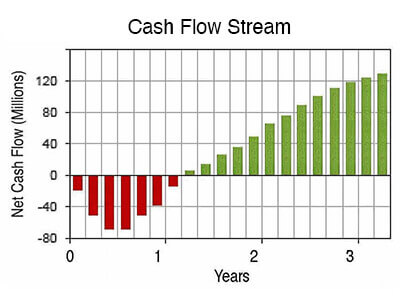Bar heidghts in this cash flow stream represent magnitudes of cash inflows and outflows. Most cash flow metrics derive directly from net cash flow magnitudes, such as these. This example is a typical investment curve profile, that is, a cash flow stream with earlier cash flows that are substantially negative and later cash flows mostly positive.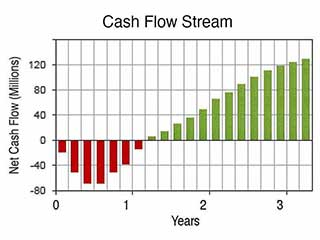Bar heidghts in this cash flow stream represent magnitudes of cash inflows and outflows. Most cash flow metrics derive directly from net cash flow magnitudes, such as these. This example is a typical investment curve profile, that is, a cash flow stream with earlier cash flows that are substantially negative and later cash flows mostly positive.

Cash flow metrics address questions like these:

• A firm expects a specific cash inflow five years from now.

Management may ask: What is the value of that future inflow today? Consequently, the present value metric provides one answer.
• An investor buys a bond, causing cash outflow from the investor's bank account. The bondholder then receives regular interest payments for the rest of the bond's life. Years later, at maturity, the investor receives a significant cash inflow.

How does a potential investor compare one such investment possibility with another? Here, the yield to maturity and return on investment metrics provide a basis for such comparisons.
• A business analyst projects a cash flow stream from an action, such as a five-year marketing campaign. And, the act brings a series of cash inflows and outflows over the years. The chart above above could represent these inflows and outflows.

How do analysts compare the financial outcomes of this action to the likely results of other acts? Cash flow metrics such as net present value and internal rate of return help make such comparisons.

### Summary: The purposes of cash flow metrics

In brief, businesspeople use cash flow metrics for the following:

• Comparing different actions or investments
• Justifying a chosen action in financial terms
• Anticipating the economic consequences of action

These uses are helpful for budgeting, planning, or decision support purposes.

## Cash Flow Metric 1 Net Cash Flow / Net CF

The net cash flow metric, not surprisingly, addresses the question: "What is the net of all cash inflows and outflows for a specific time span? Analysts usually express "Net cash flow" in currency units, as net cash flow per period. For example:

Year 1 Net Cash Flow = \$424.00

For a multi-period cash flow stream, the analyst normally calculates a net cash flow figure for each period, as well as the total net cash flow for the entire cash flow stream.

• An investment that results in positive net cash flow is a net gain. An investment resulting in a negative net cash flow is a net loss.
• Net cash flow figures do not take into account the time value of money (discounting). Net cash flow is thus insensitive to the timing of inflows and outflows within the period.
• A net cash flow figure itself does not reveal the magnitude of total inflows or total outflows. Net cash flow shows only the difference between these totals.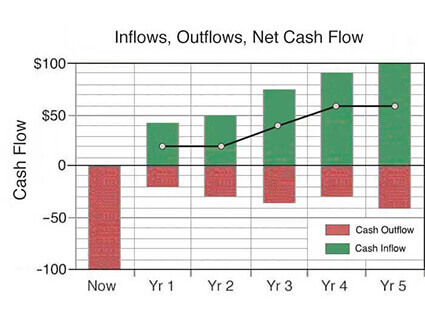The basic data for cash flow metrics are predicted cash inflows and outflows over a designated time period.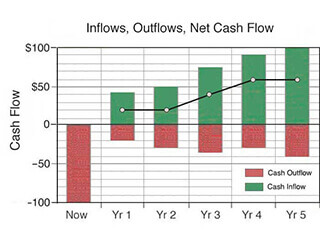The basic data for cash flow metrics are predicted cash inflows and outflows over a designated time period.

Cash Flow Metric: NET CASH FLOW

## Cash Flow Metric 2 Cumulative Cash Flow

For a series of cash flow events, the cumulative cash flow is the sum of all inflows and outflows through a specific point in time. For a multi-period cash flow stream, analysts normally calculate the cumulative cash flow value for the end point of each period. Analysts describe "Cumulative cash flow" in currency units. For example:

Cumulative cash flow at the end of Year 3 = \$1,650,000

• At the end of the final period, cumulative cash flow must equal total net cash flow for the entire cash flow stream.
• Analysts usually calculate "Cumulative cash flow" without considering the time value of money (discounting).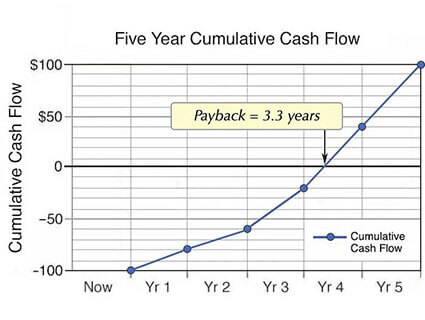Cash flow stream events plotted on a cumulative basis reveal the payback period.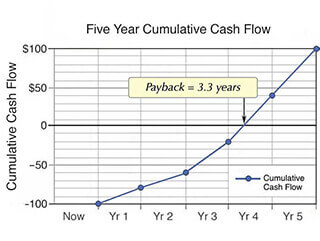Cash flow stream events plotted on a cumulative basis reveal the payback period.

Cash Flow Metric: CUMULATIVE CASH FLOW

## Cash Flow Metric 3 Future Value FV / Compound Interest

Future value FV is a cash flow metric that addresses questions like this: What will be the value after seven years of a \$1,000 bank deposit earning compound interest at a rate of 5% per year? The following FV formula answers this question:

FV5 = \$1,000 *(1.0 + 0.05)7= \$1,407

Future value (FV) is thus the value funds will have at some time in the future when they are flow in or flow out. FV in this way contrasts with the Present value metric (next section) which is instead the value today of funds to receive or pay at a future time.

Cash Flow Metric: FUTURE VALUE

## Cash Flow Metric 4 Present Value PV

Present value PV is a cash flow discounting metric that addresses questions like this: What is the value today of a \$1,000 cash inflow that is arriving seven years from now? With a discount rate (interest rate) of 5%, the PV formula answers this question:

PV7 = \$1,000 / (1.0 + 0.05)7 = \$711

The PV today (\$711) is thus discounted below the FV (\$1,000) of funds to that will flow in the future. Note that the sum of present values for a multi-period cash flow stream is a financial metric in its own right, known as net present value NPV.

The magnitude of the discounting effect for each PV depends on (1) an arbitrary interest (discount) rate, and (2) the number of discounting periods between now and the future cash flow.

• The higher the discount rate, the higher the discounting effect.
• The higher the number of discounting periods between now and actual cash flow, the higher the discounting effect.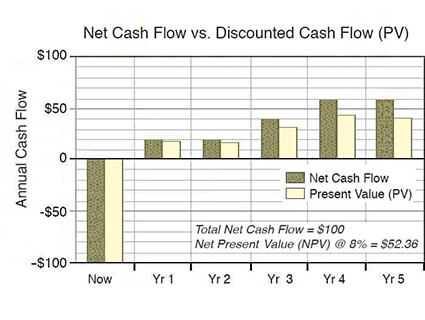Cash flow stream as net cash flow (dark bars) and as present values ( light bars).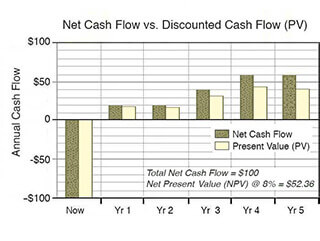Cash flow stream as net cash flow (dark bars) and as present values (discounted values, light bars).

Cash Flow Metric: PRESENT VALUE

## Cash Flow Metric 5 Payback Period

The payback period metric addresses questions like these:

• How long will it take for an investment to pay for itself?
• How long before "cumulative gains" exceed "cumulative costs?"

Analysts typically answer such questions with a figure in decimal years, for example:

Payback Period = 3.2 years

Businesspeople sometimes call Payback period the "Break-even point in time." The payback period metric derives from cumulative cash inflow and cumulative cash outflow figures, as examples show in the Payback Period. Other factors being equal, analysts view the investment with the shorter payback period as the better choice for two reasons:

• A shorter payback period means that investors recover investment costs sooner, and the funds are available again for further use.
• Analysts see the shorter payback period as less risky than a longer payback period.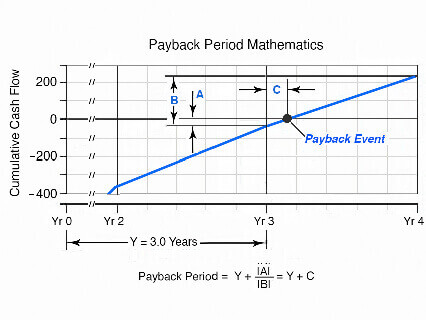Payback period is the time it takes for cumulative cash inflows to equal cash outflows, exactly.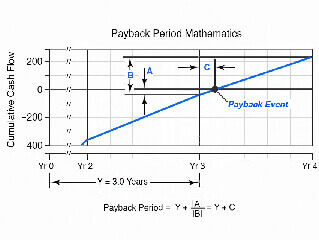Payback period is the time required for cumulative cash inflows to equal cash outflows, exactly.

Cash Flow Metric: PAYBACK PERIOD

## Cash Flow Metric 6 Return on Investment ROI

Return on Investment ROI is a favorite financial metric for evaluating the economic consequences of investments and actions. Several "metrics" carry that name, but the best known is the metric appearing in this encyclopedia as simple ROI.

Simple ROI compares returns to costs by making a ratio between cash inflows and outflows that follow from the investment. By definition, the ROI ratio calculates as net investment gains divided by total investment costs. Simple ROI in this form is legitimately called a measure of profitability.

• Analysts usually present the ROI ratio as a percentage. When the metric calculates as ROI = 0.24, for instance, the analyst reports ROI = 24.0%.
• A positive result such as ROI = 24.0% means that returns exceed costs. Analysts, therefore, consider the investment a net gain.
• The opposite kind of result, a negative ROI such as –12.7%, means that costs outweigh returns. Analysts, in that case, view the investment as a net loss.

Consequently, when comparing two or more investments—and when risks and other factors are equal—analysts consider the investment with the higher ROI to be the better choice.

Cash Flow Metric: RETURN ON INVESTMENT ROI

## Cash Flow Metric 7 Internal Rate of Return IRR

Analysts define the "Internal rate of return IRR" as the interest rate (discount rate) that yields a net present value of 0 for the net cash flow stream that follows from an investment or action. Those familiar with bond investing call the same metric (IRR) by the name yield to maturity or YTM. Investors and analysts always report IRR as a percentage, for example:

Investment IRR = 16.0%

Many businesspeople outside of finance have heard of IRR, probably because their financial officers often require an IRR estimate to support budget requests or action proposals. Some organizations define a hurdle rate as a specific IRR rate. They specify, that is, an IRR rate that incoming proposals must reach or exceed o qualify for approval and funding.

Many in the financial community believe that IRR results readily compare to inflation, current interest rates, and business investment alternatives. Note especially, however, that this belief is sometimes supportable and sometimes not. In brief:

• An IRR rate higher than the firm's cost of capital (or opportunity cost for using funds) usually means the investment should be considered a net gain.
• Other factors being equal, the investment with the higher IRR is considered a better investment.
• Further interpretation of IRR magnitudes, however, depends on (1) the firm's cost of capital, (2) the return rate the firm can earn on other invested funds, and (3) ongoing trends in rate changes.

Cash Flow Metrics: INTERNAL RATE OF RETURN IRR & MIRR

## Cash Flow Metric 8 Break Even Point

Analysts usually describe the break-even point as a particular number of units a firm produces or sells at a given price for total cash outflows to equal total cash inflows. When the unit sells more units than the break-even number, the additional units represent a net gain or positive margin.

The simple form of break-even analysis formula derives break-even quantity (Q) from just three input numbers:

P = Selling price (or Cash inflow) per unit.
v = Variable cost (the Cost added for each unit sold).
F = Overall fixed cost.

Breakeven quantity Q = B / ( Pv )

For many firms, understanding how selling price, variable cost, and fixed cost impact break-even volume is crucial in budgeting, production planning, and profit forecasting.

Cash Flow Metric: BREAK EVEN POINT

## Cash Flow Metric 9 Total Cost of Ownership TCO

Total Cost of Ownership TCO analysis attempts to uncover all the lifetime costs that follow from acquiring and owning certain assets. Not surprisingly, TCO has the name "asset life-cycle cost."

Asset ownership brings purchase costs, of course, but "ownership" also brings costs due to installing, deploying, using, upgrading, maintaining, and disposing of the same assets. These after-purchase costs can be substantial. Consequently, for many kinds of assets, TCO analysis finds a significant difference between purchase price and total life-cycle costs.

Overall TCO results appear as a single currency figure covering a designated ownership life. For example:

Estimated Five-year TCO per Vehicle = \$89,024

TCO requires the analyst to develop a comprehensive cost model—a model that identifies all the obvious costs of ownership, but also all important costs sometimes called "hidden costs." TCO results are especially useful when the analyst populates the TCO cost model with actual cost components—showing the contribution of each component to overall TCO.

Cash Flow Metric: TOTAL COST OWNERSHIP TCO

## Cash Flow Metric 10 Cumulative Average Growth RATE CAGR

Most profit-making companies are expected to grow over time—"growing" sales, profits, and shareholder value. Consequently, owners, managers, directors, employees, competitors, and industry analysts all take a keen interest in tracking business growth metrics for the company.

In this regard, multi-period growth metric cumulative average growth rate CAGR, for instance, often appears in company annual reports to address questions such as this: What was the average yearly growth rate for sales revenues across 10 years? As an example, consider a company that reported:

• First-year sales revenues (PV) of \$1,000,000.
• Eleventh-year sales revenues (FV) of \$2,593,743

The CAGR formula finds average growth rate by calculating the exponential rate that would grow the PV figure into the FV figure after ten growth increments. For this analysis, the number of growth periods, n is "10," and the CAGR formula is as follows:

CAGR = (FV / PV)(1/n) –1.0
= (\$2,593,743 / \$1,000,000)0.10 –1.0
= 1.10 –1.0 = 0.10 = 10.0% exponential growth per year

This company's annual average growth rate compares, of course, to industry averages and to competitor growth rates.

Cash Flow Metrics: GROWTH METRICS

## The Nature and Role of Financial Statement Metrics (Business Ratios)

Financial statement metrics measure the strength of a company's financial position or the company's performance over specific periods. These metrics derive primarily from figures on four financial accounting statements: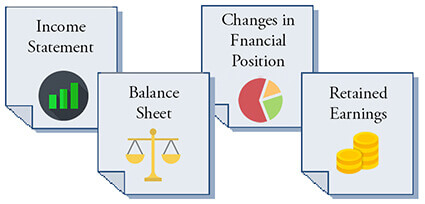Financial statement metrics (ratios) derive from numbers on the Income Statement, Balance Sheet, Statement of Changes in Financial Position, and Statement of Retained Earnings.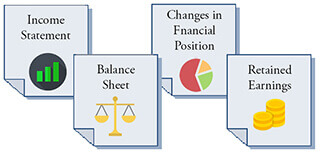Financial statement metrics (ratios) derive from numbers on the Income Statement, Balance Sheet, Statement of Changes in Financial Position, and Statement of Retained Earnings.

Financial statement metrics are generally of keen interest to:

• Investors considering buying or selling stock or bonds in a company.
• Company management, for identifying strengths, weaknesses, and target levels for business objectives.
• Shareholders and boards of directors, for evaluating management performance.

## Financial Statement Metrics /Business Ratios Group 1 Liquidity MetricsLiquidity metrics measure the organization's ability to move cash or turn other assets into cash. The label liquidity is appropriate because terms for cash movements are often terms that also describe moving water, as in cash flow, payment stream, or liquid assets

Liquidity metrics address this question primarily:

• Can the company meet its short-term financial obligations?

This encyclopedia defines, explains, and calculates the following liquidity metrics.

• Working Capital
• Current Ratio
• Quick Ratio
or Acid-Test Ratio
• APT Accounts payable turnover
• CCC Cash conversion cycle

Find input data for Liquidity Metrics in two financial reports:The primary input data for Liquidity metrics appear in the Income Statement and Balance Sheet.

Financial Statement Metrics: LIQUIDITY

## Financial Statement Metrics /Business Ratios Group 2 Activity and Efficiency MetricsActivity and efficiency metrics measure the company's run rate—turnover—for individual asset categories, such as inventories and accounts receivable.

Activity and efficiency metrics address questions like these:

• Is the company using its resources efficiently?
• Does the firm earn acceptable returns from its assets?

This encyclopedia defines, explains, and calculates the following activity and efficiency metrics.

• Sales Revenues per employee
• Inventory Turns
• Days Sales in Inventory
or Average Turnover Period
or DIO Days Inventory Outstanding
• Accounts receivable turnover
• DPO Days payable outstanding
• Average Collection Period
or DSO Days Sales Outstanding
• CCC Cash Conversion Cycle
• Total Asset Turnover
• Fixed Asset Turnover

Find input data for Activity and Efficiency Metrics in two financial reports:The primary input data for Activity and Efficiency metrics appear in the Income Statement and Balance Sheet.

## Financial Statement Metrics /Business Ratios Group 3 Leverage MetricsLeverage metrics compare funds lenders supply to the business to funds that owner/investors supply. All parties share business risks and rewards in proportion to their share of the funding.

Leverage metrics address questions like these:

• Do the company's funds come primarily from owners or from creditors?
• How do owners and creditors share business risks and rewards?

This encyclopedia defines, explains, and calculates the following leverage metrics.

• Total Debt to Asset Ratio
• Debt-to-equity ratios:
Total debt-to-equity ratio
Long-term debt to equity ratio
• Times interest earned

Find input data for Leverage Metrics in two financial reports:The primary input data for Leverage metrics appear in the Income Statement and Balance Sheet.

Financial Statement Metrics: LEVERAGE

## Financial Statement Metrics /Business Ratios Group 4 Profitability MetricsSmall business owners and large corporation shareholders, alike, take a keen interest in profitability metrics. Profitability metrics measure earning performance for a specific time period.

Profitability metrics address questions such as these:

• Is the company profitable?
• Does the firm make good use of its assets?
• Is the firm earning acceptable margins?

This encyclopedia defines, explains, and calculates the following profitability metrics.

• Profit margins:
Gross profit margin
Operating profit margin
The Net profit margin on sales (Net income)
• ROA Return on total assets
• ROCE Return on capital employed
• ROE Return on equity
Return on owners investment
RONW Return on net worth
Return on common equity
• EPS Earnings per share

Find input data for Profitbility Metrics in three financial reports: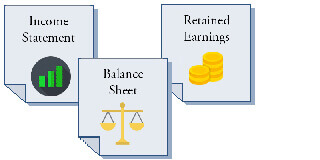The primary input data for Profitability metrics appear in the Income Statement, Balance Sheet, and Statement of Retained Earnings.

Financial Statement Metrics: PROFITABILITY

## Financial Statement Metrics /Business Ratios Group 5 Valuation Metrics

Valuation metrics address questions like this:

• What are the company's prospects for future earnings?

This encyclopedia defines, explains, and calculates the following valuation metrics.

• Earnings per share EPS
• P/E Price to earnings ratio
• Book value per share
• Market to book ratio

Find input data for Valuation Metrics in three financial reports: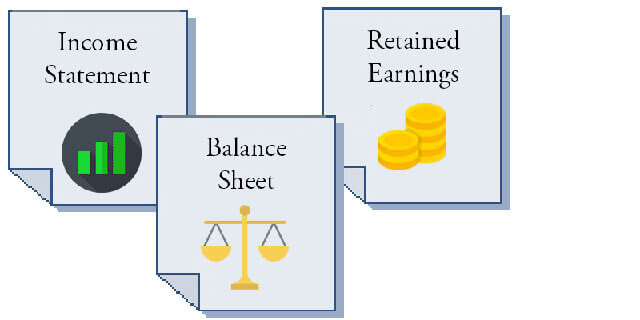The primary input data for Valuation metrics appear in the Income Statement, Balance Sheet, and Statement of Retained Earnings.

Financial Statement Metrics: VALUATION

## Financial Statement Metrics /Business Ratios Group 6 Growth MetricsEveryone with an interest in the firm regards growth metrics as indicators of the company's financial health and prospects for the future.

Growth metrics address questions like these:

• Are revenues, profits, and market share growing?
• How does the company's recent growth compare to similar companies?
• How does the firm's historical growth compare to industry averages?

This encyclopedia defines, explains, and calculates the following growth metrics:

Find input data for Growth Metrics from multi-period results appearing in three financial reports:The primary input data for Valuation metrics appear in the Income Statement, Balance Sheet, and Statement of Retained Earnings.

Financial Statement Metrics: GROWTH